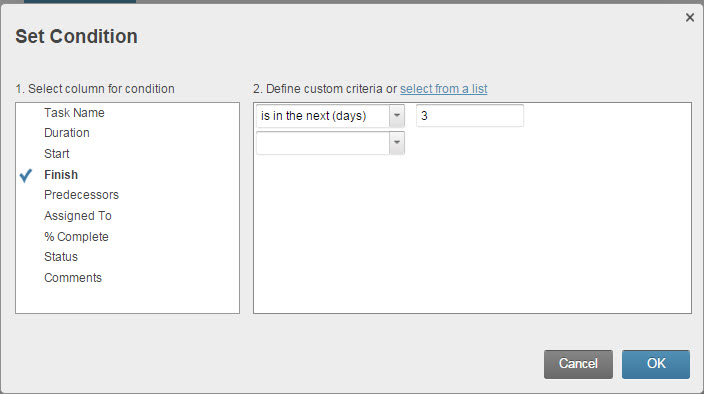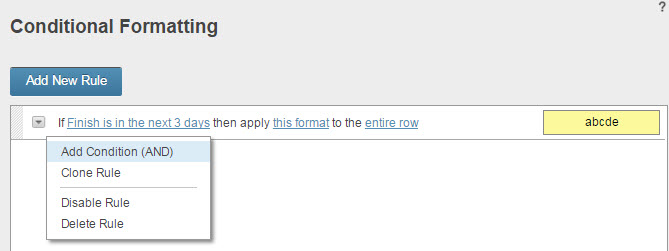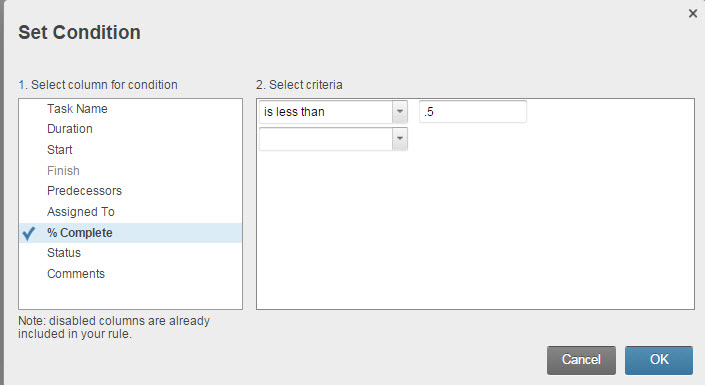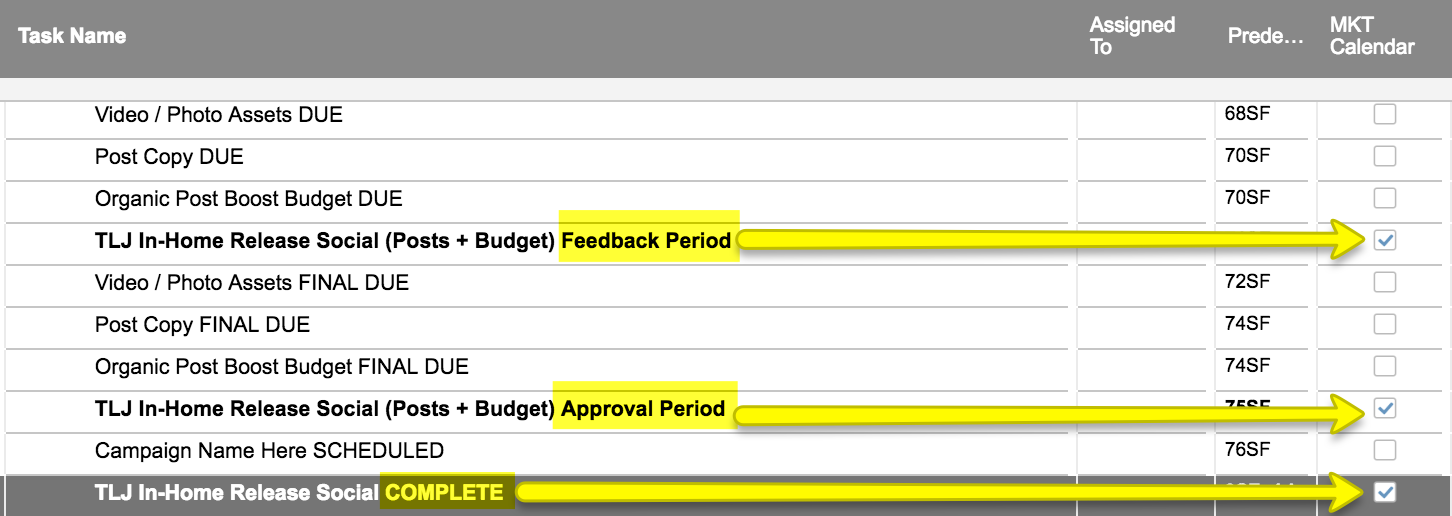#### Welcome to the Smartsheet Forum Archives

The posts in this forum are no longer monitored for accuracy and their content may no longer be current. If there's a discussion here that interests you and you'd like to find (or create) a more current version, please Visit the Current Forums.

# Checkbox Checked depending on text

Should be simple, but I'm struggling.

Two columns

Text Column that contains some text.

Checkbox Column

I would like a folmula for the checkbox column to check the text column for some text.  If the text column contains the text, mark the checkbox checked.  Can this be done?

• Put in a simple IF statment in the checkbox column,

Column2 is a text column

Column3 is a checkbox column

This IF statement checks the box if column2 contains "test"

=IF([Column2]1 = "test", 1, 0)

• Hello there, yes an IF statement is what you want to do and Ian gave a good example.

You can also tell it to check if greater than zero because the greater than works for both numbers and text.  So it could look like:

=IF(Column2 > 0, 1, 0)

This will allow you to put any text you want in the column and it will check the box rather than looking for specific text. However, I would use Ian's example if I wanted specific text to populate the checkbox.

• Another way to look for ANY text is to use the ISTEXT function:

=IF(ISTEXT(Column2), 1)

Or to check if the cell is blank (will uncheck if blank):

=IF(ISBLANK(Column2), 0, 1)

• Thank you everyone.

• Actually that did not work.

=IF([Column2]1 = "test", 1, 0) only checks the box if the text is exactly "test".  I am looking for something that checks the box if the text in the textBox INCLUDES "test".

For example if we had the formula looking to the word test, the following would check the box:

1. Test
2. Testing
3. Tested
4. Test fire rocket

...

• This is probably what you want then:

=IF(FIND("test", [Column2]1) >= 1, 1, 0)

if it finds any instance of "test" it checks the box

• OK - I'm doing the same here.  My issue is that if the box is checked per the formula (all works correctly) but then I decide I don't want it check and manually undo it, then the formula is erased.

Checkbox named "At Risk"   Formula to show that it might be at risk so a human can review it.  But if he human overrules it and unchecks it, then the formula disappears.

Formula in use for the checkbox.

=IF((TODAY() - Finish26) < 3, AND([% Complete]26 < "50%")), 1, 0)

Any ideas?

• Yes.

Unfortunately, manual entry will ALWAYS overwrite the formula.

What I have done in these situations, is apply conditional formatting instead.

Example:

Row background = green IF((TODAY() - Finish26) < 3, AND([% Complete]26 < "50%")), 1, 0)

• Well, crap.  Thanks for the quick response, though!

• ACharvet.

How do I apply that to a cell?  Conditional Formatting doesn't seem to accept formulas.

•• Hi - not sure if this will go unanswered since this thread is from a few years ago, but I have a question that takes the same subject matter of the topic here one step further.

How would I adjust this formula =IF(FIND("test", [Column2]1) >= 1, 1, 0) to include an OR function.

The use case I'm trying to accomplish is check the box if:

• A row contains "Feedback Period" (but has variable text within it as well)
• A row contains "Approval Period" (but has variable text within it as well)
• A row contains "COMPLETE" (but has variable text within it as well)

An example of my sheet with the desired outcome (hacked manually for now) is attached.

Appreciate any help that can be offered!This discussion has been closed.